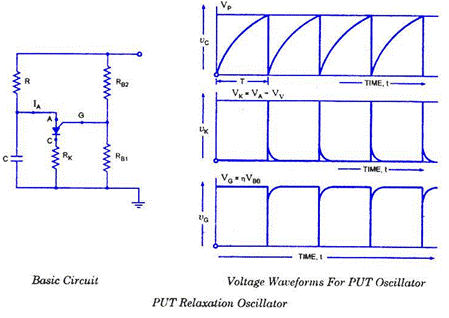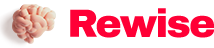# Non-linear or relaxation oscillator and operational amplifier relaxation oscillator

SaveSavedRemoved 0
Deal Score0
Deal Score0

The relaxation oscillator is a repetitive circuit that realizes the repetitive behavior from the capacitor charging to the threshold event. This event discharges the capacitor, and its recharge time verifies the repetition time of the event. In a simple flasher circuit, the battery charges a capacitor through a resistor, and the value of the resistor and capacitor at a constant time determines the flash rate. The flicker rate can be maximized by reducing the resistance value.

Author: Andrew Carter

The relaxation oscillator is a repetitive circuit that realizes the repetitive behavior from the capacitor charging to the threshold event. This event discharges the capacitor, and its recharge time verifies the repetition time of the event. In a simple flasher circuit, the battery charges a capacitor through a resistor, and the value of the resistor and capacitor at a constant time determines the flash rate. The flicker rate can be maximized by reducing the resistance value.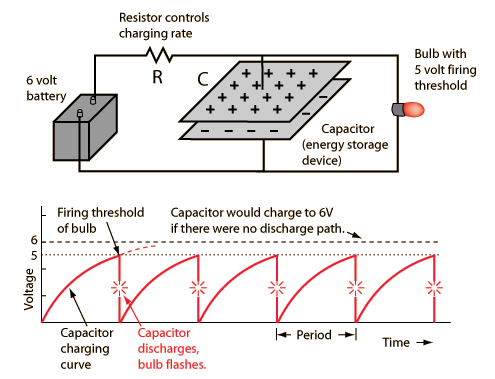The very popular 555 integrated circuit has to be a relaxation oscillator.It can detect voltage, And operate the transistor switch to discharge the capacitor when the voltage is reached, Turn it off again when the voltage drops.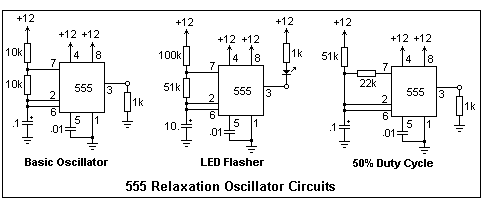Op amp relaxation oscillator

The link below shows a common op amp relaxation oscillator. The non-inverting input is biased at V/2, where V is the output voltage. The inverting input follows this value, and when it reaches this value, the output voltage changes from one saturation level to the opposite saturation level. If the saturation level is symmetrical, the output is a square wave with a frequency of 1/1.22RC and a duty cycle of 50%.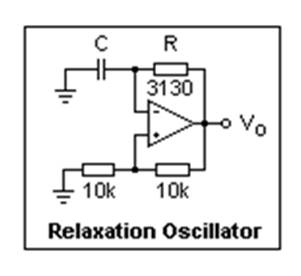Sample question:

For the op amp relaxation oscillator given below, calculate the values ​​of R and C to achieve 23kHz oscillation.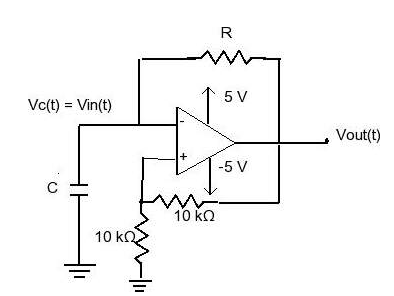solution:

The oscillation frequency is given by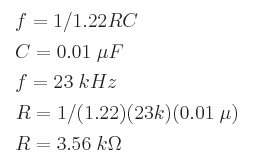Single junction transistor relaxation oscillator

A single junction transistor or UJT is a switching device, not an amplifier.It is similar to JFET in that it is an N-type silicon rod, both ends are related, called(B1) and(B2), there is a PN junction in the middle, and P silicon is connected to the emitter (E) end. The current usually flows from B2 to B1, forming a potential gradient along the rod. Therefore, as long as the PN junction is reverse biased, the current generated through the external resistor R will produce a voltage spike, as shown in the figure, the capacitor voltage drops to the value V. Then the device is switched off and the capacitor starts to charge again. This cycle repeats continuously, producing a sawtooth waveform on capacitor C.

Programmable unijunction transistor relaxation oscillator

Programmable unijunction transistor (PUT) is a four-layer PNPN device that can be arranged very much like UJT. The electrodes are anode (A), cathode (K) and grid (G), and the symbols are the same as SCR. The voltage VS is applied to the upper N zone, which usually reverse biases the middle PN junction, so no current flows from the anode to the cathode. PUT is called programmable because the voltage can be chosen to be equivalent to changing the interval ratio η of UJT.